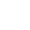# 數算日子的智慧：貝氏統計學家的婚姻難題

2019/10/29 | | 標籤：

Dear Abby 是 1956 年開始發行、流傳甚廣的美國顧問專欄，起初的作者 Pauline Phillips 已在 2013 年過世，現由她女兒繼續以同名執筆經營。Dear Abby 經常為讀者提供諮商，為他們解決各種疑難雜症。下面這封讀者來函曾被列入統計學教科書裡，我也常用來作為基本統計學的教材。

「Dear Abby：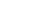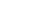Pr(產期≥11/7|受孕期=1/1)= 0.003

Pr(受孕期=1/1|產期≥11/7)=？

1. 老婆在 1 月 1 日之後，是否有出軌受孕的機會？假設真正的受孕期是 1 月 1 日之後的第X天。X=0 代表老婆沒出軌，受孕期真的是 1 月 1 日；X>0代表老婆在 1 月 1 日後出軌才受孕。
2. 自己一向對老婆有多少信心？依自己的主觀判斷，老婆未出軌，即 X=0 的機率有多少？假設 X=0 的機率為Y，X>0的機率為 1-Y，則 Y 越接近 1 信心越高，越接近0信心越低。Y是X=0的邊際機率，1-Y是X>0的邊際機率。這邊際機率也就是貝氏定理所謂的先驗機率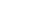D≥310-X 的機率顯然與X有關，我們用p(X)來代表此一條件機率：p(X)=Pr(D≥310-X|X)。因為懷孕時間呈常態分配：D~N(266,162)，我們可以導出：

$$p\left ( X \right )= Pr\left ( D\geq 310- X|X \right )= \frac{1}{2}Erf\left ( \frac{44-X}{16 \sqrt{2}} \right )$$

 「行」的條件機率 老婆 1/1後未出軌：X=0 （受孕期=1/1） 老婆 1/1 後出軌：X>0 （受孕期=1/1 後第X天） D<310-X（產期<11/7） 1-p(0) 1-p(X) D≧310-X（產期≧11/7） p(0) p(X) 「行」的邊際機率 Y 1-Y

 聯合機率 老婆 1/1後未出軌：X=0 （受孕期=1/1） 老婆 1/1 後出軌：X>0 （受孕期=1/1 後第X天） D<310-X（產期<11/7） Y(1-p(0)) (1-Y)(1-p(X)) D≧310-X（產期≧11/7） Y(p(0)) (1-Y)(p(X)) 「行」的邊際機率 Y 1-Y

Pr(受孕期 =1/1 │產期≧11/7)$$=Pr\left ( X=0 | D\geq 310-X\right )$$ $$= \frac{Y p(0)}{Y p(0)+(1-Y)p(X)}$$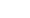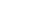《可能性調查署2》上線囉！接下來每周都會有新影片，快去按讚訂閱開啟小鈴鐺

## 關於作者